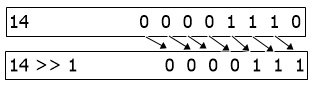# Bitwise Operators with Example

Bitwise operators are used for bitwise manipulation. That is, bitwise operators operate on binary integers and units. That is, whatever the operand value is, the bitwise operator performs the calculation on its binary equivalent's values.

## Types of Bitwise Operators

These are the six types of bitwise operators:

Now that we've gone over all of the bitwise operators, let's go over each one in greater detail and understand it using an example that's both concise and easy to grasp.

## Bitwise AND (&)

The truth table for the bitwise AND or bitwise & operator is as follows:

A B A & B
1 1 1
1 0 0
0 1 0
0 0 0

For example:

```  0011 1100   (60)
& 0000 1101   (13)
-----------
0000 1100   (12)```

Therefore, 60 & 13 = 12.

## Bitwise OR (|)

The truth table for the Bitwise OR or Bitwise | operator is as follows:

A B A | B
1 1 1
1 0 1
0 1 1
0 0 0

For example:

```  0011 1100   (60)
| 0000 1101   (13)
-----------
0011 1101   (61)```

Therefore, 60 | 13 = 61.

## Bitwise XOR (^)

The truth table for the Bitwise XOR or Bitwise ^ (also known as Bitwise Exclusive OR) is as follows:

A B A ^ B
1 1 0
1 0 1
0 1 1
0 0 0
```  0011 1100   (60)
^ 0000 1101   (13)
-----------
0011 0001   (49)```

Therefore, 60 ^ 13 = 49.

## Bitwise Not (˜)

The Bitwise NOT or Bitwise ˜ operator, unlike other Bitwise operators, is an unary operator.A unary operator is one that only operates on one operand or takes one input.

The bitwise NOT operator complements each bit of input one by one. We can understand "complement" here as reverse. The following is the truth table for the Bitwise, NOT operator:

A ˜A
1 0
0 1

For example:

```˜ 0111   (7)
------
1000   (8)```

Therefore, ˜7 = 8.

## Bitwise Right Shift (>>)

The Bitwise Right Shift, or Bitwise >> operator, shifts the binary sequence to the right. For example, the binary equivalent of 14 is 0000 1110 (8-bit binary equivalent), and then 14 >> 1 gives 000 0111. The graphic representation of the Bitwise right-shift operation is shown below:Note: Empty places get replaced by 0. As a result, 14 >> 1 = 0000 0111 (which 7).

Similarly, 14 >> 2 can be calculated as:

```14            0000 1110
14 >> 2         00 0011```

Therefore, 14 >> 2 = 0000 0011 (which equals 3). That is, the binary equivalent of 14 is shifted two bits to the right.

## Bitwise Left Shift (<<)

The Bitwise Left Shift, or Bitwise << operator, shifts the binary sequence to the left. For example, the binary equivalent of 14 is 0000 1110 (8-bit binary equivalent), and then 14 << 1 gives 0001 1100. The figure given below shows, in graphical form, how the bitwise left shift operation performs:Note: In bitwise left shift, empty places also get replaced by 0. Therefore, 14 << 1 = 0001 1100 (that is equal to 28).

Similarly, 14 << 2 can be calculated as:

```14            0000 1110
14 << 2       0011 10```

Therefore, 14 << 2 = 0011 1000 (that is equal to 56).

Computer Fundamentals Quiz

« Previous Tutorial Next Tutorial »